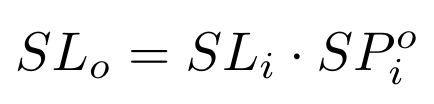# Impermanent Loss and Slippage for Weighted Pools

Weighted Math is designed for Weighted Pools to allow for swaps between any assets whether or not they have any price correlation. Prices are determined by the pool balances, pool weights, and amounts of the tokens that are being swapped.

## Invariant

The value function
$V$
is defined as:
$V= \prod_t B_t^{W_t}$
Where
• $t$
ranges over the tokens in the pool
• $B_t$
is the balance of the token in the pool
• $W_t$
​is the normalized weight of the tokens, such that the sum of all normalized weights is

## Value of Weighted pools

A weighted pool can be comprised of up to 8 different ERC20 tokens, each with its own arbitrary weight. The weights represent the percentage of value that the pool should be holding in each token at any time. That percentage of value the pool holds in each token is expected to be always very close to the pool weight, so long as there are rational arbitrageurs active in the market and the pool fee is low.
Some key questions one can ask when providing liquidity to a weighted pool are:
• Is there a way to generalize the calculation of how much a weighted pool is worth after the prices of underlying tokens all changed?
• How much will a pool that holds 75% AVAX and 25% USDC grow in value if AVAX's price doubles and USDC stays constant?
• What is the loss of a pool if a token with 10% weight goes down by 5x?
A very simple formula offers the answer to all these questions. The value of a weighted pool in any reference (for example USD) changes according to the price changes in that same reference (USD) of each of its underlying tokens: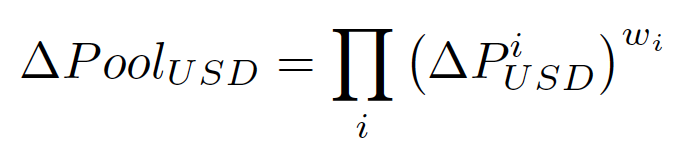where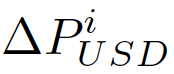is the variation in USD price of token i, and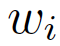is the weight of token i.
With this formula at hand, we can easily answer the questions we posed above. Notice that token prices that don’t change don’t affect the pool value (ΔP_usd^w = 1^w = 1), whatever the weight of those tokens. So we have:
• If AVAX's price doubles, a pool with 75% AVAX and 25% USDC will have a total value increase of 68.2% (=2^0.75=1.682) as opposed to 41.4% (=2^0.5=1.414) for a 50/50 pool.
• A pool that has a token with a weight of 10% that goes down by 5x (an 80% crash, or ΔP_usd = 0.2) will have a total value decrease of only about 15% (=0.2^0.1=0.8513).

## Impermanent Loss

If you never heard the term before, please take a read at his important article about returns on Uniswap pools.
Impermanent Loss Simulator:
By definition, impermanent loss (IL) describes the percentage by which a pool is worth less than what one would have if they had instead just held the tokens outside of the pool. In other words: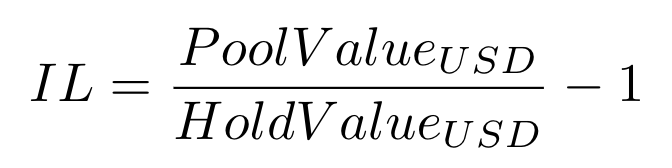This formula considers a theoretical pool with 0% fee. Hexagon's Value Function without fees is path independent (that is, the order of trades does not change the final state of the pool). This implies that, if the liquidity provider removes their liquidity when all the relative token prices are the same as when they added liquidity, the impermanent loss will be zero: they will have exactly the same amount of tokens they've invested.
Adding fees to the equation, the final profit in USD terms by the liquidity provider will be the accumulated fees in terms of % of total pool value subtracted by the impermanent loss.
We can expand the formula for impermanent loss above using the following definition for variation of hold value for the pool: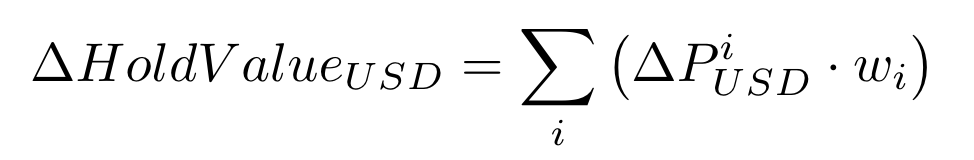To clarify this formula, imagine, for example, a holding basket that has a token with a weight of 50% and that token doubled in USD value. The total value of the holding basket will increase by 50%, or by 1.5x (=1 * 50% + 2 * 50% = 1.5). In other words, imagine initially holding $500 in token A and$500 in token B, a total of $1000. If token B doubles in value you'll then be holding$500 in token A and $1000 in token B, a total of$1500 (1.5x or 50% more, as expected).
As detailed in the article mentioned above, impermanent loss can also be written in terms of the variation in pool value and hold value: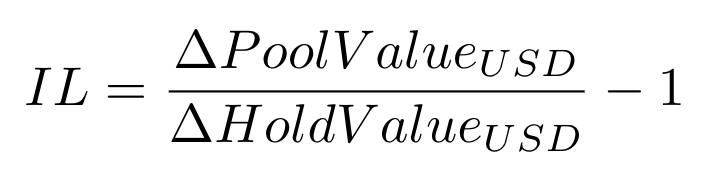So replacing the formulas above we get to our final expression for impermanent loss in weighted pools: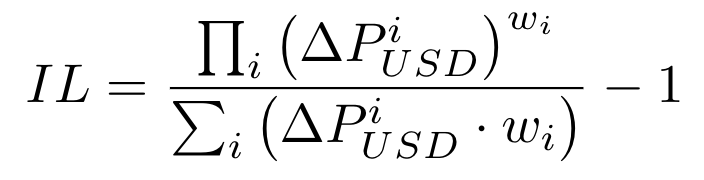Notice that because of the convexity of the Value Function, the impermanent loss will always be either zero or a negative number. This could lead to confusion with a negative loss being interpreted as a gain. This is not the case: the real meaning of the IL being always negative is that there is always some impermanent loss with any relative change in token prices.

## Slippage in Weighted Pools

By definition, slippage is the percentage change in the effective price paid in a trade relative to the spot price. The spot price is defined as the limit of the effective price as the amount traded tends to zero.
In weighted pools with a fee, the spot price is defined by (notice that from here on i means the input token, i.e. the token that is being sent/sold to the pool by the trader):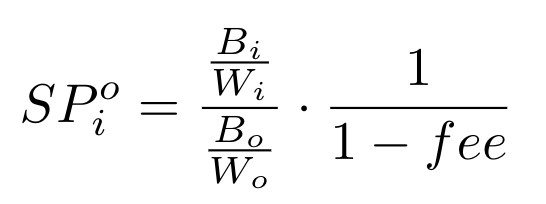The effective price of a trade is by definition the ratio of the amount the trader sold (amountIn) divided by the amount of the other token they got in return (amountOut):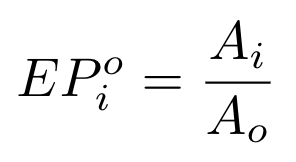Using the formula for amountOut we can rewrite this as: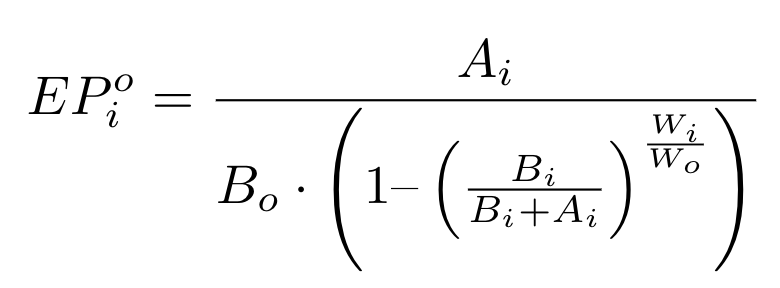Note that, even though we are dealing with trades where the user defines the amount they want to sell (amountIn), the whole derivation below can be done for when the user defines the amount they want to buy (amountOut), as detailed in the following section.
We can define slippage as the percentage by which the effective price exceeds the spot price. It's a function of the amountIn traded (Ai) as it influences the effective price: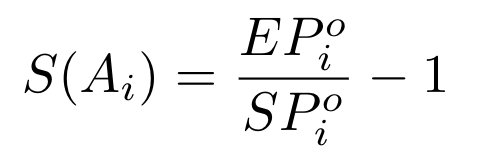So if the effective price of a trade is 102 and the spot price is 100, then the slippage is 2%.
Slippage is not linear: it grows faster with increasing amounts being traded. As an approximation though, we can linearize the slippage for small values traded. The chart below shows the effective price for increasing amounts sold in blue and the linearization of the effective price with slippage in orange (all numbers are fictitious):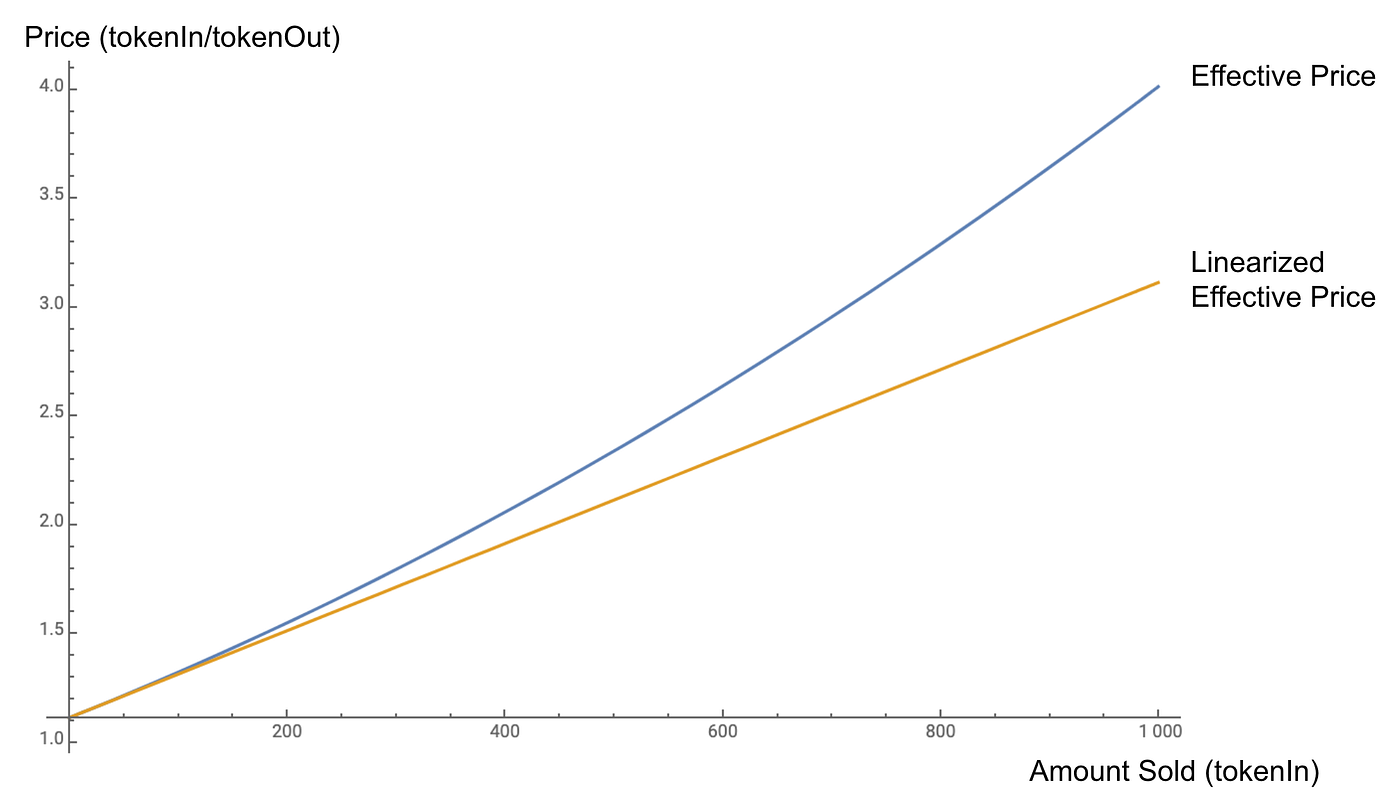This linearization is extremely useful for many algorithmic optimizations.
By linearizing we can rewrite the slippage as a linear function of the amount traded: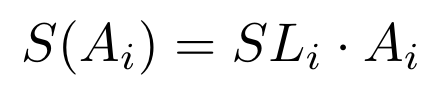The slippage slope (SL) is simply the derivative of the slippage formula defined above at Ai = 0. This can be calculated in different ways, but the final solution is: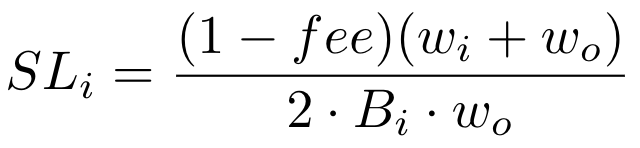### Slippage for AmountOut

For completeness's sake, let's derive the formula for the slippage given the amount bought of a token, amountOut (Ao). The formulas are very similar, we start from the same effective price formula:But this time around we expand Ai as a function of Ao, as derived on our whitepaper: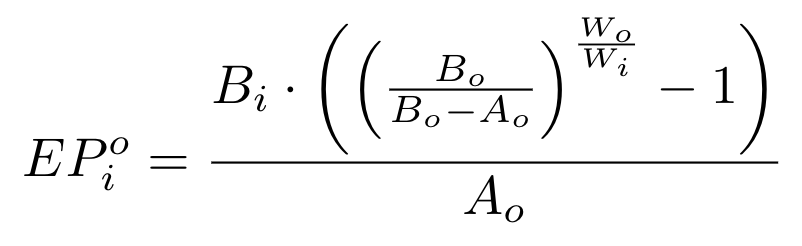And the slippage can be written as a function of Ao: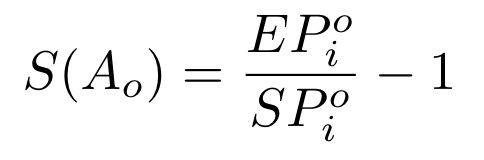Linearizing: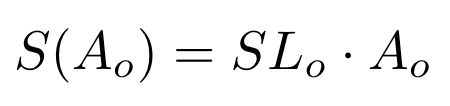Solving the derivative we have: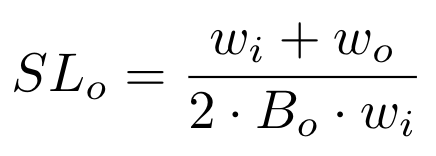It's interesting to notice that, as one would expect, the slippage is symmetric in a pool. That is, if one trades a small amount relative to the size of the pool, it should not matter in which direction (from token A to B or from token B to A) the trade is: the slippage in % should be the same for the same value being traded. This can be observed as the slippage slopes calculated for Ao and for Ai are the same in terms of % per value traded, they are only in different units. SLi is % per Ai traded and SLo is % per Ao traded: